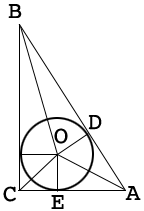SEARCH HOMEMath Central Quandaries & QueriesQuestion from Daksh: O is the centre of the inscribed circle in a 30°-60°-90° triangle ABC right angled at C. If the circle is tangent to AB at D then the angle COD is-Hi,

I can help get you started.

Since the circle is tangent to the sides of the triangle at $D$ and $E,$ angles $ADO$ and $OEA$ are right angles.What can you say about triangles $ADO$ and $OEA?$

PennyMath Central is supported by the University of Regina and The Pacific Institute for the Mathematical Sciences.